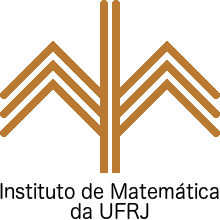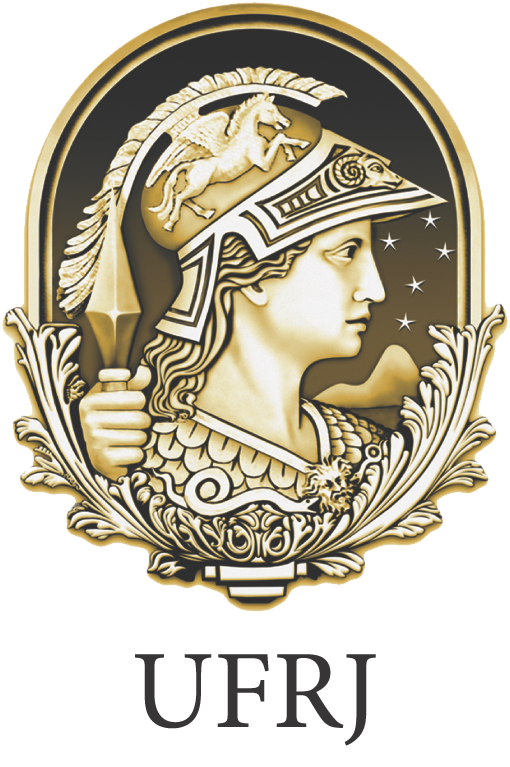# Teaching

• Calculus of one and several variables

• Linear Algebra

• Differential equations

• Mathematical Modelling

• Real Analysis

• Complex variables

• Measure theory

• Functional analysis

• Semigroups

• Navier-Stokes equations and turbulence

## Short-courses

• "Resultados recentes sobre as equações de Navier-Stokes para fluidos incompressíveis". Primeira Escola Brasileira de Equações Diferenciais (I EBED), IMECC-Unicamp, Campinas, SP. June 9-13, 2003. Slides

• "Equações de Navier-Stokes e turbulência". Summer Program at LNCC, Petrópolis, RJ. February 24-27, 2003. Slides

• "Equações de Navier-Stokes e turbulência". Summer Program at IM-UFRJ, Rio de Janeiro, RJ. January 4, 6, 8, 18, 20, 22, 2002.

• "Navier-Stokes equations and the statistical theory of turbulence", Seventh Paseky School on Mathematical Theory in Fluid Mechanics, Paseky, Czech Republic. June 3-10, 2001.

• "Sistemas dinâmicos em dimensão infinita". Short course at IM-UFRJ, Rio de Janeiro, RJ. July 1995.